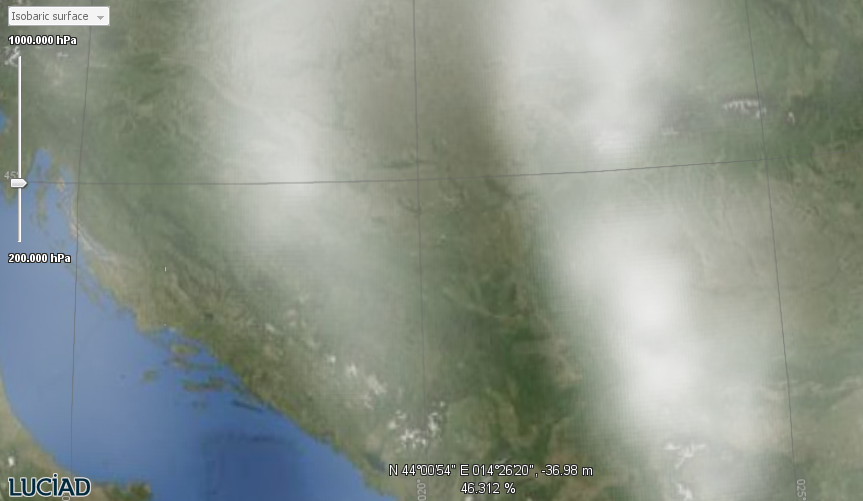Many NetCDF models contain a set of measurement grids, each of which is associated with a particular point in time and/or a vertical position. To visualize a particular measurement grid, the NetCDF model is able to filter itself. `TLcdNetCDFFilteredModel` allows querying the dimensions that can be filtered, and configuring a parameter or interval for every dimension, time and altitude for example, to filter out the desired measurement grids. You can filter out the measurement grid captured at an altitude of 827 meters on January 20, for instance.

Usually the end users of an application decide which particular measurement grids they are interested in, by making a selection in the graphical interface. In such a case, the filter is configured by a GUI selection widget. The NetCDF sample includes a filter UI called `FilterManagerUI` for this purpose. The panel retrieves the set of available vertical positions and/or the set of points in time from the `TLcdNetCDFFilteredModel`. You can see how this is done in Program: Retrieving the dimension axes and data ranges of a multi-dimensional NetCDF model for a filter GUI..

Program: Retrieving the dimension axes and data ranges of a multi-dimensional NetCDF model for a filter GUI. (from `samples/common/dimensionalfilter/model/FilterProvider`)
``````ILcdMultiDimensionalModel filteredModel = (ILcdMultiDimensionalModel) model;
List<? extends ILcdDimension<?>> dimensions = filteredModel.getDimensions();
if (dimensions != null) {
for (ILcdDimension dimension : dimensions) {
if (shouldHandleDimensionAxis(dimension)) {
DimensionalFilter dimensionalFilter = createFilter(aLayered, aLayer, dimension);
}
}
}``````

Program: Interactively filtering a multi-dimensional NetCDF model. shows how to change the filtering based on a filter GUI change event.

Program: Interactively filtering a multi-dimensional NetCDF model. (from `samples/common/dimensionalfilter/model/FilterProvider`)
``````ILcdMultiDimensionalModel model = (ILcdMultiDimensionalModel) getLayer().getModel();
try (TLcdLockUtil.Lock ignored = TLcdLockUtil.writeLock(model)) {
TLcdDimensionInterval<?> newValue = TLcdDimensionInterval.createSingleValue(
type,
aValue
);
TLcdDimensionFilter filter = TLcdDimensionFilter.newBuilder().filterDimension(fDimension.getAxis(), newValue).build();
TLcdDimensionFilter snappingFilter = filter.createSnappingFilter(model, TLcdDimensionFilter.SnapMode.NEAREST);
List<TLcdDimensionInterval> values = getDimension().getValues();
if (!values.get(0).isSingleValue()) {
//get the missing dimension values from snapping filter
for (TLcdDimensionAxis axis : snappingFilter.getAxes()) {
if (!axis.equals(getDimension().getAxis())) {
filter = filter.asBuilder().filterDimension(axis, snappingFilter.getInterval(axis)).build();
}
}
} else {
filter = snappingFilter;
}
model.applyDimensionFilter(filter, ILcdModel.FIRE_LATER);
} finally {
getLayer().getModel().fireCollectedModelChanges();
}``````Figure 1. NetCDF humidity data filtered at a specific atmospheric pressure level